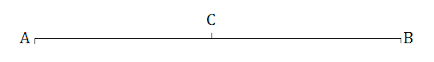Newbie

# If a point C lies between two points A and B such that AC = BC, then prove that AC = ½ AB. Explain by drawing the figure.Q.4

• 0

Find this important question of ncert class 9th of chapter Introduction To Euclid’s Geometry.Sir please help me to find out the easiest and simplest solution of question 4 of exercise 5.1, give me the best solution of this question.If a point C lies between two points A and B such that AC = BC, then prove that AC = ½ AB. Explain by drawing the figure.

Share

1. Solution:Given that, AC = BC

L.H.S+AC = R.H.S+AC

AC+AC = BC+AC

2AC = BC+AC

We know that, BC+AC = AB (as it coincides with line segment AB)

∴ 2 AC = AB (If equals are added to equals, the wholes are equal.)

⇒ AC = (½)AB.

• 0